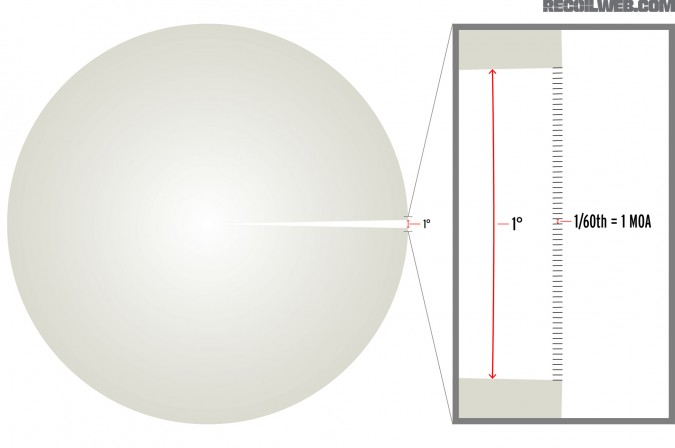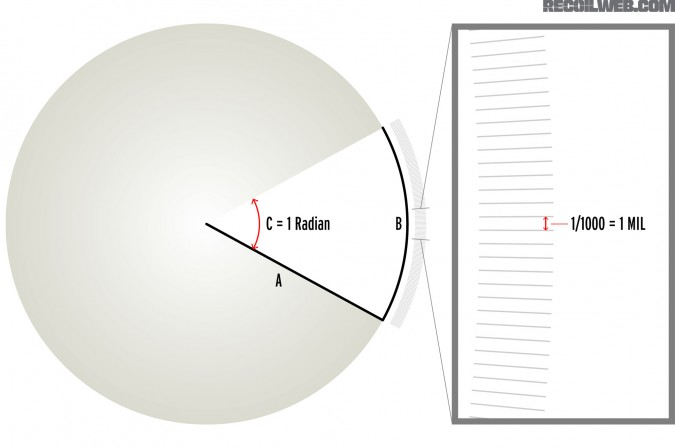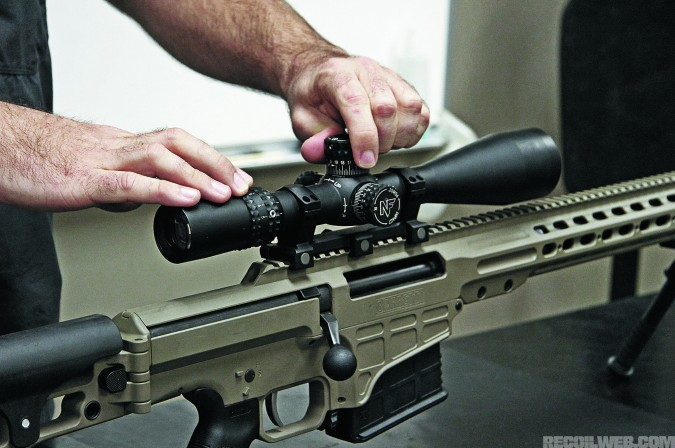The Ultimate Firearms Destination for the Gun Lifestyle

MOA vs Mil

BEAR WITH US, ‘CAUSE IT’S TIME TO GET YOUR MATH ON

The argument over whether to use Minutes of Angle (MOA) or milliradians (mil) is one that’s guaranteed to spark a lively online debate among shooters.

Before you get sucked into the fray, arm yourself with a healthy understanding of the difference between the two systems, and how to make them work for you.

Both MOA and mils are units of angular measurement. In shooting, angular measurements are used to describe linear size, relative to distance (e.g. 10 inches tall at 300 yards, or 15 centimeters left at 200 meters). The most common uses are incremental adjustments to a bullet’s impact, estimating the distance of a known-size target, “holding” for windage or elevation, and measuring accuracy by shot-group size.

The most important thing to understand about these measurements is that they are angular! For example, when we adjust our scopes we move the reticle inside the scope, which then forces us to move the barrel of the rifle up, down, left, or right in order to get the reticle back on to the target. This difference between where the rifle’s barrel was pointed prior to an adjustment in windage or elevation and where the barrel is pointed after the adjustment can be measured as a change in angle. A certain angular adjustment translates into smaller changes in the bullet’s impact at closer distances and larger changes at further distances.

To help you understand how an angular measurement translates into different sizes at different distances, imagine holding two laser pointers next to each other and pointing them down range. If you spread the two laser pointers apart at a certain angle, the lasers would gradually get further and further apart from each other as they went down range. For any given angle, however, the rate at which the dots spread apart is consistent — the dots will be twice as far apart at 200 yards and 10 times as far apart at 1,000 yards as they were at 100 yards. See Figure 1.

MINUTE OF ANGLE (MOA)

In the term Minute of Angle, the word minute means 1/60th (for example, 1 minute of time is 1/60th of one hour) and the word angle refers to one of the 360 degrees in a circle. So, 1 Minute of Angle is 1/60th of a degree. (See Figure 2.)If we spread the two laser pointers from the example above apart 1 MOA (1/60th of a degree), then the two lasers’ dots would be about 1 inch apart at 100 yards, about 2 inches apart at 200 yards, about 3 inches apart at the 300 yards and so on. Simply stated, this means that 1 Minute of Angle is about 1 inch per 100 yards. I say “about” 1 inch because 1 MOA is actually 1.047 inches per 100 yards, but using 1 inch per 100 yards is close enough for our purposes. Cue the slapfest. Many will argue that it’s crucial to use 1.047 inches per 100 yards, and if you don’t it’ll make the baby Jesus cry.

I don’t disagree that it’s more accurate; it’s just that in the real world I don’t notice enough of a difference to use the more accurate number, but hey, maybe you do. Let me qualify that. At 1,000 yards, when I use 1 inch per 100 yards, 1 MOA equals 10 inches. When 1.047 inches per 100 yards is used, 1 MOA equals 10.47 inches. The 0.47 inch difference at 1,000 yards is barely wider than the width of my .308 Win bullet and is so small that you can’t even adjust for it on a scope (the finest most scopes adjust is ½ MOA, which is about 2.5 inches at 1,000 yards). Therefore, even if I needed to move my bullet’s impact up 11 inches at 100 yards, the closest adjustment I can make is up 1 MOA.

Where things get hinky though, is if you try to convert drop values in inches to MOA, if these are coming from a source other than your own DOPE cards. Let’s say you have a ballistic app or a store-bought “ballistic card” that say you need to come up XX inches at a certain distance. For example, if your information says that you’ll need 425 inches of elevation at 100 yards, you’d convert that into 42.5 MOA using 1 inch per 100 yards or 40.5 MOA using 1.047 inches per 100 yards. That 0.47-inch difference is repeated 42 times for a total difference of 19.74 inches, or 2 MOA. At this point, those who are fixated on the 1.047 value will yell, “Aha! Gotcha.”

Whatever. It’s still almost totally irrelevant. First, reference data from another source is likely not to be perfect for your system because of different environmental effects and differences in internal ballistics. And second, because most shooters prefer to gather their own data on previous engagements and would rather not to have to drag around a calculator in the field, they’re going to stick to 1 inch per 100 yards and do just fine.

Just as the meter is the metric equivalent of the yard for distance, the milliradian is the metric equivalent of the MOA for angular measurement.

In the term milliradian, the prefix milli- means 1/1,000th and the root radian is the metric unit of angular measurement. So, 1 milliradian is 1/1,000th of a radian. (See Figure 3.)A radian is an angle based on a circle’s radius, or half of its diameter. When the length of a section of a circle equals the radius of that circle, the resulting angle is a radian. In simpler terms, if you placed a string, which is the same length as the radius (“A” in Figure 3), along the edge of the circle (“B”), the angle formed from the center of the circle to each end of the string would be one radian (“C”). Now, if you divided that angle into 1,000 equal smaller angles, one of those smaller angles would be one milliradian.

This is where the simplicity of using milliradians comes in. If you imagine yourself standing at the center of the circle in Figure 3 and you spread two laser pointers 1 milliradian apart and shine them along the radius to the edge of the circle (“A” distance), then the dots will spread exactly 1/1,000th of the length of the radius (“A” distance) apart.

I have heard many people argue over whether 1 milliradian equals 1 meter at 1,000 meters or whether it equals 1 yard at 1,000 yards. They are both right! One milliradian equals 1/1,000th of any distance. It is 1 inch at 1,000 inches and 1 mile at 1,000 miles. It does not matter what unit of measurement you are using so long as you keep using that unit of measurement. Of course, it’s easier to use mils with meters though, because it’s easier to calculate 1 centimeter (cm) is 1/100th of a meter than it is to calculate 0.36 inch is 1/100th of a yard.

USING MOA AND MILS

When learning how to use MOA or mils, always think in whole increments for a particular distance. What I mean is this. Start with the target’s distance and ask yourself how big 1 MOA or 0.1 mil is at that distance. Then, determine how many of those “chunks” you need to adjust.

For example, with MOA, if your target is 600 yards away, you should first calculate that 1 MOA is about 6 inches and then ask yourself how many 6 inch “chunks” you need to adjust your bullet’s impact. If you need to move 3 inches at 600 yards, that is ½ of a 6-inch “chunk” so you need to move ½ MOA. Likewise, if you need to move 12 inches at 600 yards, that is two 6-inch “chunks” so you need to move 2 MOA.

With mils, if your target is 500 meters away, you should first calculate that 0.1 mil is 5 centimeters (or 1 mil is 0.5 meters) and then ask yourself how many 5cm “chunks” you need to adjust the bullet’s impact.CONVERTING BETWEEN MOA AND MILS

Approximately 3.5 MOA equal 1 mil. To be accurate, there are two different precise conversions, because there is a difference between a true milliradian and a NATO milliradian. The number of true milliradians in a circle is not an even number (it’s actually 6,283.18…) and it is not easily divided into equal parts. Because of this, many militaries have rounded the number up to 6,400. Therefore, there are 6,283 true milliradians and 6,400 NATO milliradians in a circle. One true mil equals 3.438 MOA and 1 NATO mil equals 3.375 MOA, but don’t let this take you off into the weeds.

To convert from mils to MOA, multiply the mils by 3.5. To convert from MOA to mils, divide the MOA by 3.5.

Mils x 3.5 = MOA

As with use of 1 inch per 100 yards for MOA, it’s not necessary to use the precise conversion from MOA to mils. First off, when I am converting between the two, precision is generally not required. For example, when I was first learning to read wind I’d calculate the wind hold in MOA and then convert to mils so that I could use the reticle in my scope, (thanks, Big Army!) Generally, a wind call, especially while you’re first learning, isn’t precise enough to have the difference between 3.375 or 3.5 matter. Also, using the precise figure for MOA and mil just doesn’t make much of a difference.

For example, with my rounding of 1 inch per 100 yards for MOA and 3.5 MOA to 1 mil, I calculate that 1 mil equals about 3.5 inches at 100 yards. Now, if I use the precise numbers of 1.047 inches per 100 yards for MOA and 3.375 MOA to 1 mil, I calculate that 1 mil equals precisely 3.53 inches at 100 yards. By rounding, I was able to calculate approximately 3.5 inches in my head quickly, instead of a precise 3.53 inches slowly. The difference at 100 yards is 0.03 inches. Even at 10 times that distance, the difference is only 0.3 inch. Leave the arcane hair-splitting arguments for the chairborne rangers on Internet forums to worry about — we’ll be off shooting stuff.

WHICH IS BETTER?

My simplest advice is to use whatever you are most comfortable with. If you normally think in inches and yards and have experience with MOA then you might want to stick with MOA. If, however, you are comfortable with the metric system or are starting fresh and don’t already have MOA experience, you might want to go with mils. Contrary to what you might hear on the range, mils are not more precise than MOA. In fact, mil scopes generally can only adjust in 1/10 mil increments, while MOA scopes often adjust in ½ MOA increments.

Regardless of which unit of measurement you choose, pick a scope that uses the same unit for its turrets and reticle. Newer scopes are available in mil/mil and MOA/MOA combinations that are much easier to use than a scope with turret adjustments in MOA and a reticle with marks in mil. Something else to consider is what unit your peers will be using. Regardless of my personal preference, I’d rather use whatever my shooting buddies use so that we can speak the same language.